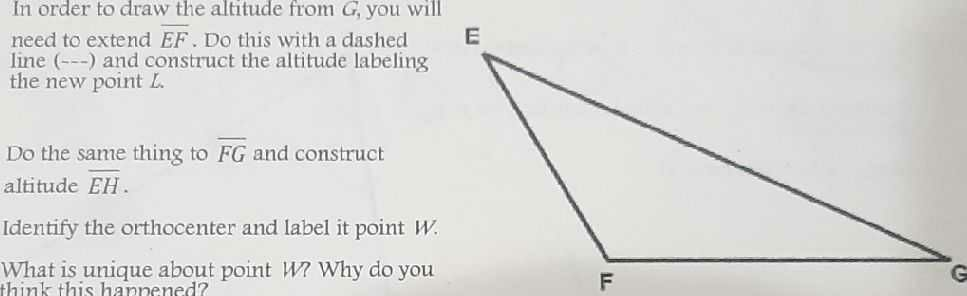### Still have math questions?In order to draw the altitude from $$G$$ , you will need to extend $$\overline { E F }$$ . Do this with a dashed line $$( - \ - \ - )$$ and construct the altitude labeling the new point $$L$$ . Do the same thing to $$\overline { F G }$$ and construct altitude $$\overline { E H }$$ . Identify the orthocenter and label it point $$W$$ . What is unique about point $$W ?$$ Why do you think this happened?